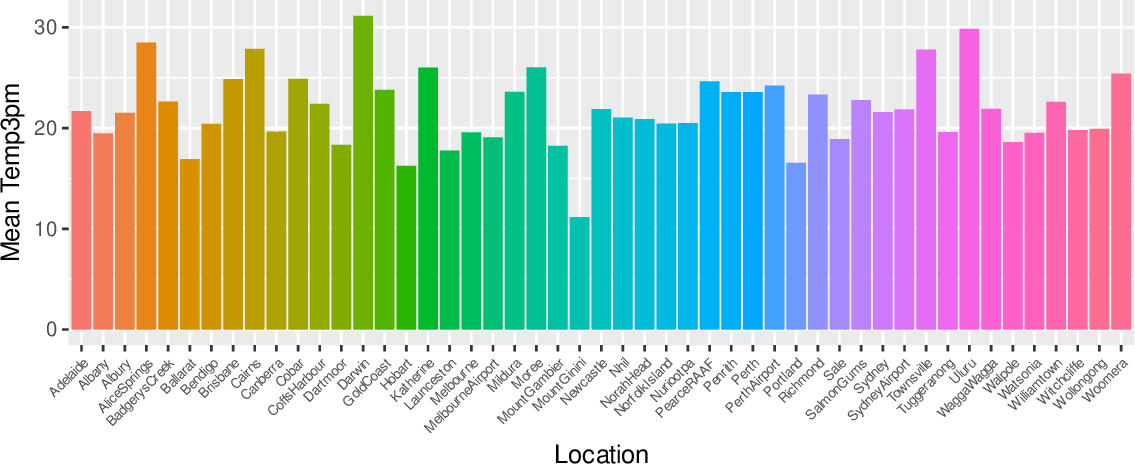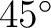Data Science Desktop Survival Guide by Graham WilliamsDesktop Survival Project Home Preface Data Science Introducing R R Constructs R Tasks R Strings R Read, Write, and Create Data Template Data Exploration Data Wrangling Data Visualisation Statistics ML Template ML Scenarios ML Activities ML Applications ML Algorithms Cluster Analysis Decision Trees Computer Vision Graph Data Privacy Literate Data Science Coding with Style Resources Bibliography Index

## Labels Rotated

20200428ds %>%   ggplot(aes(x=location, y=temp_3pm, fill=location)) +   stat_summary(fun="mean", geom="bar") +   labs(x=vnames["location"],        y=glue('Mean {vnames["temp_3pm"]}')) +   theme(legend.position="none",         axis.text.x=element_text(angle=45, hjust=1, size=6)) When a bar chart has many bars and non-trivial labels then it is useful to rotate the labels. Here we plot the mean temperature at 3pm for each location in the dataset. Without rotating the x-axis labels (the locations) the x-axis will be blighted with much overlapping. Labels can be rotated using a ggplot2::theme() option of axis.text.x= with angle=. Use hjust=1 to align the labels appropriately.